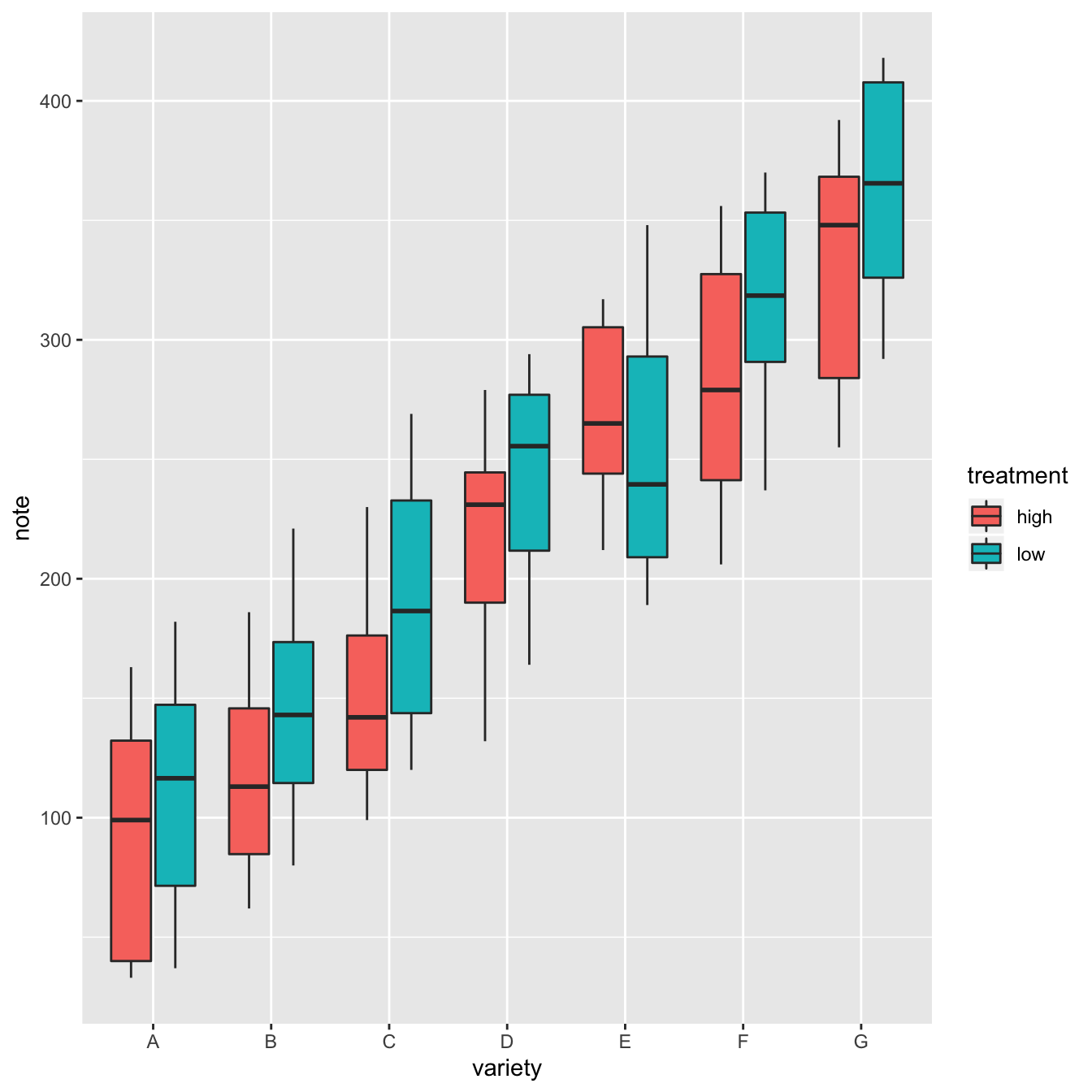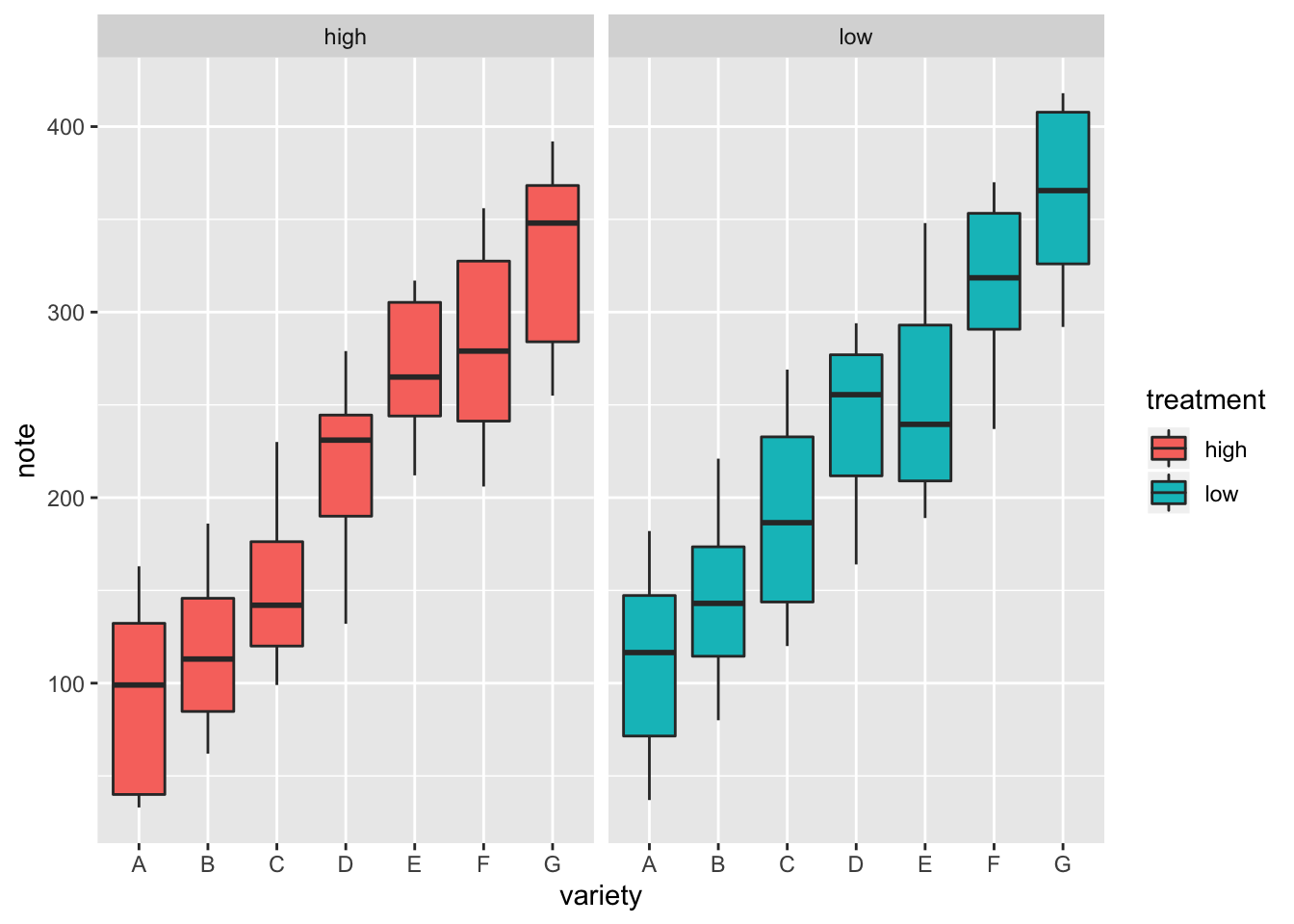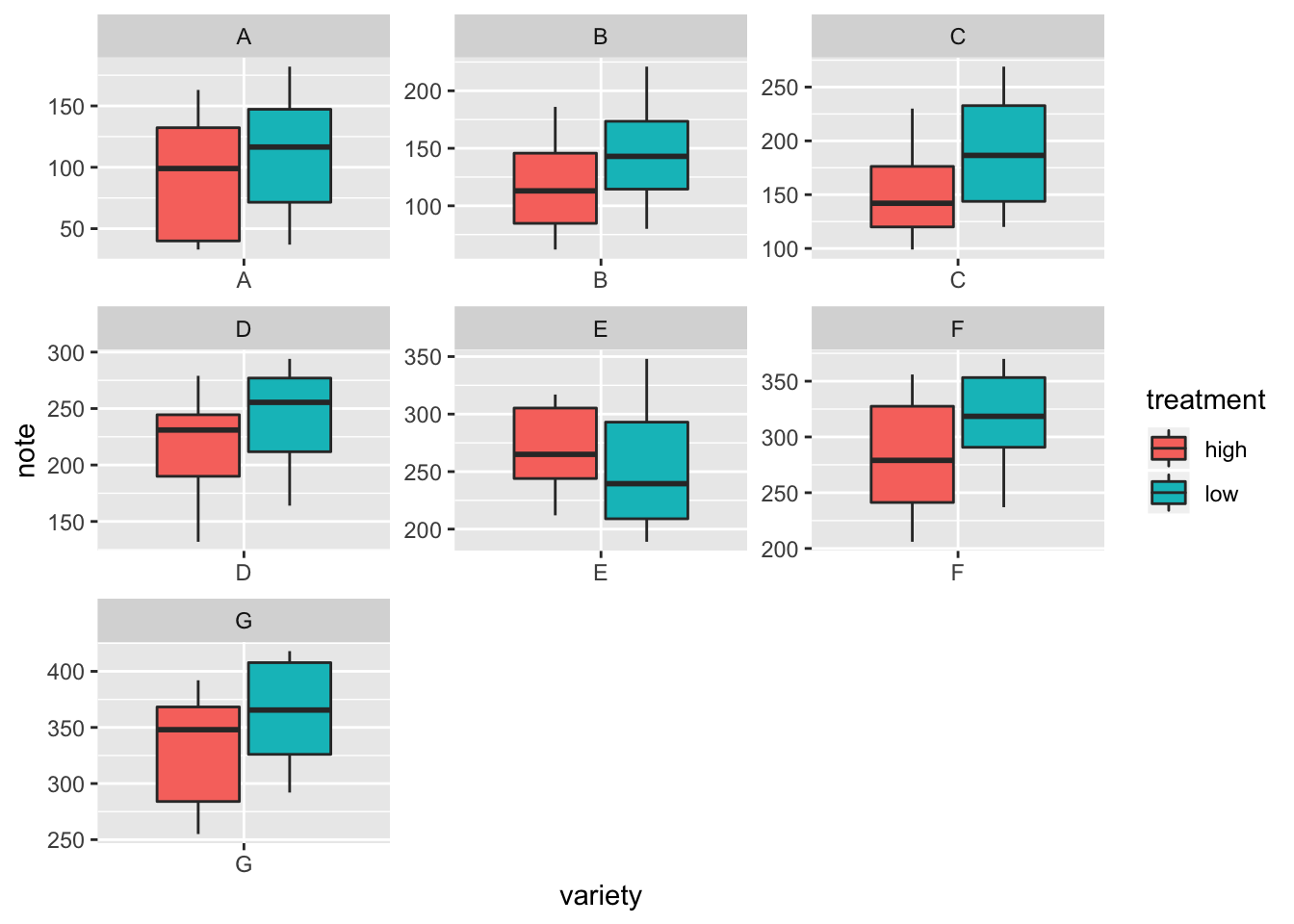# Grouped boxplot with ggplot2

A boxplot summarizes the distribution of a continuous variable for several categories. If categories are organized in groups and subgroups, it is possible to build a grouped boxplot. Here is an example with R and ggplot2.

# Grouped boxplot

A grouped boxplot is a boxplot where categories are organized in groups and subgroups.

Here we visualize the distribution of 7 groups (called A to G) and 2 subgroups (called low and high). Note that the group must be called in the `X` argument of `ggplot2`. The subgroup is called in the `fill` argument.``````# library
library(ggplot2)

# create a data frame
variety=rep(LETTERS[1:7], each=40)
treatment=rep(c("high","low"),each=20)
note=seq(1:280)+sample(1:150, 280, replace=T)
data=data.frame(variety, treatment ,  note)

# grouped boxplot
ggplot(data, aes(x=variety, y=note, fill=treatment)) +
geom_boxplot()``````

# Using small multiple

Note that an alternative to grouped boxplot is to use faceting: each subgroup (left) or each group (right) is represented in a distinct panel.

``````# One box per treatment
p1 <- ggplot(data, aes(x=variety, y=note, fill=treatment)) +
geom_boxplot() +
facet_wrap(~treatment)
# one box per variety
p2 <- ggplot(data, aes(x=variety, y=note, fill=treatment)) +
geom_boxplot() +
facet_wrap(~variety, scale="free")``````Related chart types

## Contact

This document is a work by Yan Holtz. Any feedback is highly encouraged. You can fill an issue on Github, drop me a message on Twitter, or send an email pasting yan.holtz.data with gmail.com.